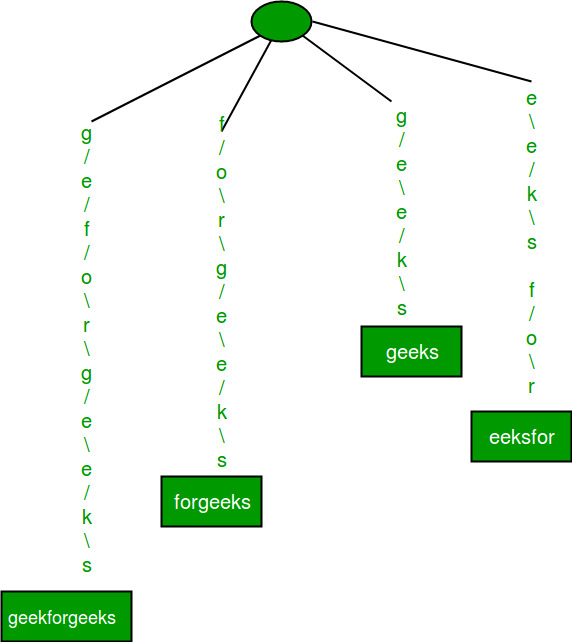Related Articles
Prefix matching in Python using pytrie module
• Last Updated : 23 Nov, 2020

Given a list of strings and a prefix value sub-string, find all strings from given list of strings which contains given value as prefix ?

Examples:

```Input : arr = ['geeksforgeeks', 'forgeeks',
'geeks', 'eeksfor'],
prefix = 'geek'
Output : ['geeksforgeeks','geeks']
```

## Recommended: Please try your approach on {IDE} first, before moving on to the solution.

A Simple approach to solve this problem is to traverse through complete list and match given prefix with each string one by one, print all strings which contains given value as prefix.

We have existing solution to solve this problem using Trie Data Structure. We can implement Trie in python using pytrie.StringTrie() module.

### Create, insert, search and delete in pytrie.StringTrie() ?

• Create : trie=pytrie.StringTrie() creates a empty trie data structure.
• Insert : trie[key]=value, key is the data we want to insert in trie and value is similar to bucket which gets appended just after the last node of inserted key and this bucket contains the actual value of key inserted.
• Search : trie.values(prefix), returns list of all keys which contains given prefix.
• Delete : del trie[key], removes specified key from trie data structure.Note : To install pytrie package use this pip install pytrie –user command from terminal in linux.

 `# Function which returns all strings ``# that contains given prefix ``from` `pytrie ``import` `StringTrie `` ` `def` `prefixSearch(arr,prefix): ``     ` `    ``# create empty trie ``    ``trie``=``StringTrie() `` ` `    ``# traverse through list of strings ``    ``# to insert it in trie. Here value of ``    ``# key is itself key because at last ``    ``# we need to return ``    ``for` `key ``in` `arr: ``        ``trie[key] ``=` `key `` ` `    ``# values(search) method returns list ``    ``# of values of keys which contains ``    ``# search pattern as prefix ``    ``return` `trie.values(prefix) `` ` `# Driver program ``if` `__name__ ``=``=` `"__main__"``: ``    ``arr ``=` `[``'geeksforgeeks'``,``'forgeeks'``,``'geeks'``,``'eeksfor'``] ``    ``prefix ``=` `'geek'``    ``output ``=` `prefixSearch(arr,prefix) ``    ``if` `len``(output) > ``0``: ``        ``print` `(output) ``    ``else``: ``        ``print` `(``'Pattern not found'``)`

Output:

`['geeksforgeeks','geeks']`

This article is contributed by Shashank Mishra (Gullu). If you like GeeksforGeeks and would like to contribute, you can also write an article using contribute.geeksforgeeks.org or mail your article to contribute@geeksforgeeks.org. See your article appearing on the GeeksforGeeks main page and help other Geeks.